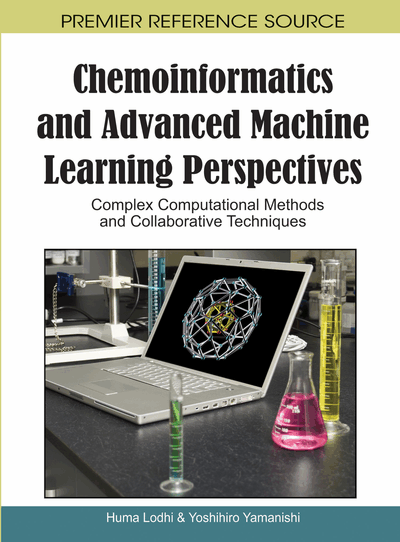# Graph Kernels for Chemoinformatics

Hisashi Kashima (University of Tokyo, Japan), Hiroto Saigo (Kyushu Institute of Technology, Japan), Masahiro Hattori (Tokyo University of Technology, Japan) and Koji Tsuda (AIST Computational Biology Research Center, Japan)
DOI: 10.4018/978-1-61520-911-8.ch001

## Abstract

The authors review graph kernels which is one of the state-of-the-art approaches using machine learning techniques for computational predictive modeling in chemoinformatics. The authors introduce a random walk graph kernel that defines a similarity between arbitrary two labeled graphs based on label sequences generated by random walks on the graphs. They introduce two applications of the graph kernels, the prediction of properties of chemical compounds and prediction of missing enzymes in metabolic networks. In the latter application, the authors propose to use the random walk graph kernel to compare arbitrary two chemical reactions, and apply it to plant secondary metabolism.
Chapter Preview
Top

## Introduction

In chemoinformatics and bioinformatics, it is effective to automatically predict the properties of chemical compounds and proteins with computer-aided methods, since this can substantially reduce the costs of research and development by screening out unlikely compounds and proteins from the candidates for ‘wet” experiment. Data-driven predictive modeling is one of the main research topics in chemoinformatics and bioinformatics. A chemical compound can be represented as a graph (Figure 1) by considering the atomic species (such as C, Cl, and H) as the vertex labels, and the bond types (such as s (single bond) and d (double bond)) as the edge labels. Similarly, chemical reactions can be analyzed as graphs where the chemical compounds and their relationships during the reaction are considered as vertices and edges, respectively (Figure 3). Prediction based on such graph representations by using machine learning techniques will help discover new chemical and biological knowledge and lead in designing drugs. However, it is not obvious how to apply machine learning methods to graph data, since ordinary machine learning methods generally assume that the data is readily represented as vector structures called feature vectors.

Figure 3.

The reaction graph for the reaction Loganin+NADPH+H++Oxygen<=>Secologanin+NADP++H2O, which is catalyzed by secologanin synthase (EC 1.3.3.9). Edges except for ‘main’, ‘cofactor’ are all ‘group’ edges in this reaction

Recently, kernel methods (Bishop, 2006, Shawe-Taylor & Cristianini, 2004) have attracted considerable attention in the field of machine learning and data mining, since they can handle (non-vectorial) structural data as if it was represented as vectorial data through the use of kernel functions. In the framework of the kernel methods, various kernel functions have been proposed for different types of structured data, such as sequences (Lodhi, Saunders, Shawe-Taylor, Cristianini, & Watkins, 2002, Leslie, Eskin, & Noble, 2002, Leslie, Eskin, Weston, & Noble, 2003), trees (Collins & Duffy, 2002, Kashima & Koyanagi, 2002), and graphs (Kashima, Tsuda, & Inokuchi, 2003, Gärtner, Flach, & Wrobel, 2003). They have been successfully applied to tasks in various areas including bioinformatics and chemoinformatics (Schölkopf, Tsuda, & Vert, 2004).

In the first part of this chapter, we introduce two approaches to graph data analysis, the pattern-based methods and the kernel methods, and then give a brief introduction to the kernel methods. We introduce one of the state-of-the-art graph learning methods called the random walk graph kernel (Kashima et al., 2003, Gärtner et al., 2003) and its extensions. The random walk graph kernel uses random walks on two given labeled graphs to generate label sequences, and the two graphs are compared based on the label sequences. Although the number of possible label sequences is infinite, we introduce an efficient algorithm for computing the kernel function without an explicit enumeration of the label sequences.

The second part of this chapter is devoted to applications of the random walk graph kernel. First, we introduce an application to predict the properties of chemical compounds. We use two data sets, one for predicting the carcinogenicity of chemical compounds, and the other for predicting whether or not each of the given compounds has mutagenicity. The results show that the graph kernel is comparable to a pattern-based method.

## Complete Chapter List

Search this Book:
Reset